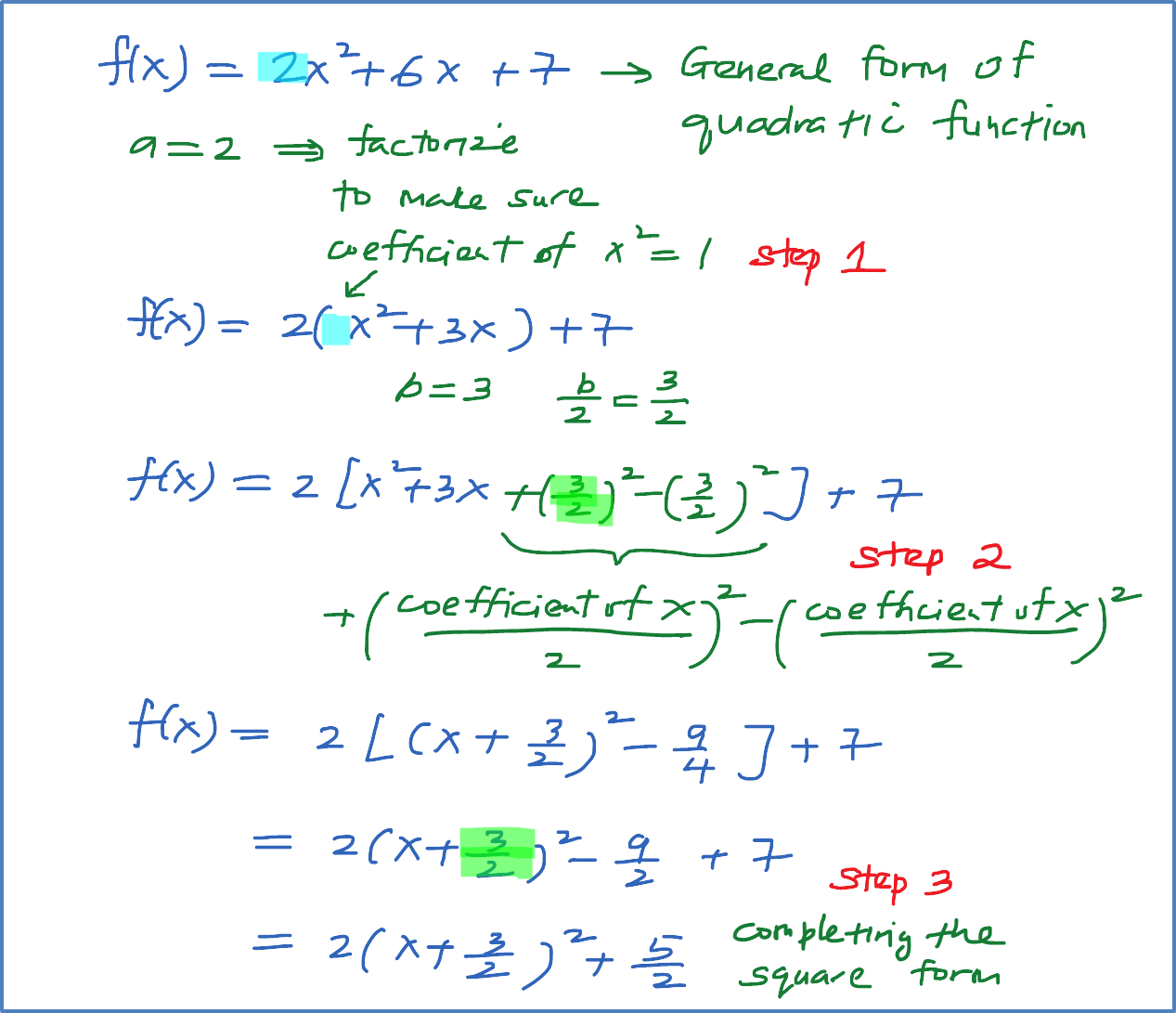\

# 2.10.2 Finding the Maximum and Minimum points of Quadratic Function using completing the square

Steps to convert general form of Quadratic Function into completing the square form

General form of quadratic function : $f\left(x\right)=a{x}^{2}+bx+c$

Completing the square form : $f\left(x\right)=a{\left(x+p\right)}^{2}+q$

Step 1 : Make sure the coefficient ${x}^{2}$ is 1, if not factorize.
Step 2 : Insert $+{\left(\frac{coefficient\text{​}\text{​}-of\text{​}x}{2}\right)}^{2}-{\left(\frac{coefficient\text{​}\text{​}-of\text{​}x}{2}\right)}^{2}$
Step 3 : Completing the square [convert $f\left(x\right)=a{x}^{2}+bx+c$ into $f\left(x\right)=a{\left(x+p\right)}^{2}+q$ .]